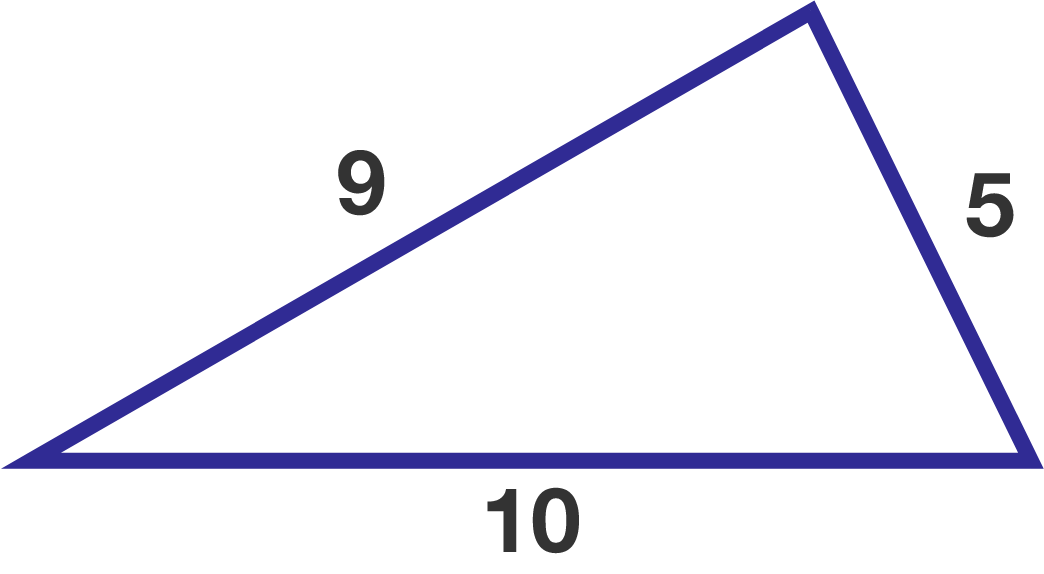# Inscribed perimeter

Geometry Level 3

Suppose you inscribe a smaller triangle in the triangle below such that its three vertices are on different sides of the given triangle.

What is the smallest possible perimeter of the inscribed triangle?×